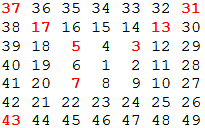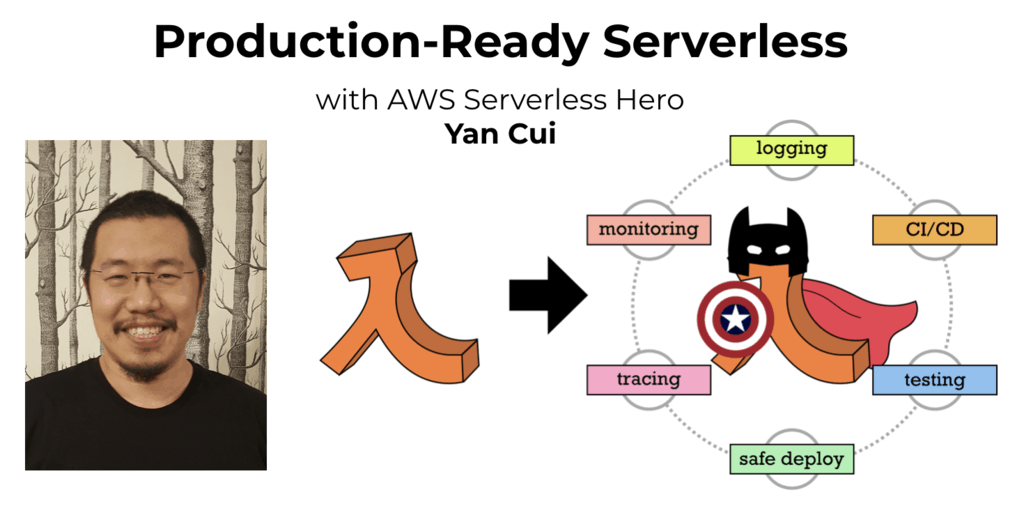# Project Euler – Problem 58 Solution

Check out my new course Learn you some Lambda best practice for great good! and learn the best practices for performance, cost, security, resilience, observability and scalability.

#### Problem

Starting with 1 and spiralling anticlockwise in the following way, a square spiral with side length 7 is formed.It is interesting to note that the odd squares lie along the bottom right diagonal, but what is more interesting is that 8 out of the 13 numbers lying along both diagonals are prime; that is, a ratio of 8/1362%.

If one complete new layer is wrapped around the spiral above, a square spiral with side length 9 will be formed. If this process is continued, what is the side length of the square spiral for which the ratio of primes along both diagonals first falls below 10%?

#### Solution

let hasDivisor(n) =
let upperBound = int(sqrt(double(n)))
[2..upperBound] |> Seq.exists (fun x -> n % x = 0)

let isPrime(n) = if n = 1 then false else not(hasDivisor(n))

// define function that returns the number on the corners of a spiral of length n
let getCornerNumbers n =
match n with
| 1 -> 
| _ when n % 2 = 0 -> []
| _ -> [3..-1..0] |> List.map (fun n’ -> n*n – n’*(n-1))

let mutable cornerNumbers, primeNumbers, size = 0, 0, 1
let mutable continueLoop = true

while continueLoop do
// get the numbers that appear at the corners of a spiral of the given size
let newNumbers = getCornerNumbers size

// increment the totals
cornerNumbers <- cornerNumbers + newNumbers.Length primeNumbers <- primeNumbers + (newNumbers |> List.filter isPrime |> List.length)

let ratio = double(primeNumbers) / double(cornerNumbers)
if ratio < 0.1 && size > 1 then continueLoop <- false else size <- size + 2 size [/code] UPDATE: Having stumbled upon some very good algorithms for generating prime number sequence here, I decided to revisit my solution here and using the PGSimple3 algorithm the new code now runs in seconds!

// generate all prime numbers under <= this max let max = 100000 // initialise the list with 2 which is the only even number in the sequence let mutable primeNumbers =  // only check the prime numbers which are <= the square root of the number n let hasDivisor n = primeNumbers |> Seq.takeWhile (fun n’ -> n’ <= int(sqrt(double(n)))) |> Seq.exists (fun n’ -> n % n’ = 0)

// only check odd numbers <= max let potentialPrimes = Seq.unfold (fun n -> if n > max then None else Some(n, n+2)) 3

// populate the prime numbers list
for n in potentialPrimes do
if not(hasDivisor n) then primeNumbers <- primeNumbers @ [n] // use the same hasDivisor function instead of the prime numbers list as it offers // far greater coverage as the number n is square rooted so this function can // provide a valid test up to max*max let isPrime n = if n = 1 then false else not(hasDivisor(n)) // define function that returns the number on the corners of a spiral of length n let getCornerNumbers n = match n with | 1 -> 
| _ when n % 2 = 0 -> []
| _ -> [3..-1..0] |> List.map (fun n’ -> n*n – n’*(n-1))

let mutable cornerNumbers, primeNumbers, size = 0, 0, 1
let mutable continueLoop = true

while continueLoop do
// get the numbers that appear at the corners of a spiral of the given size
let newNumbers = getCornerNumbers size

// increment the totals
cornerNumbers <- cornerNumbers + newNumbers.Length primeNumbers <- primeNumbers + (newNumbers |> List.filter isPrime |> List.length)

let ratio = double(primeNumbers) / double(cornerNumbers)
if ratio < 0.1 && size > 1 then continueLoop <- false else size <- size + 2 size [/code]Hi, I’m Yan. I’m an AWS Serverless Hero and the author of Production-Ready Serverless.

I specialise in rapidly transitioning teams to serverless and building production-ready services on AWS.

Are you struggling with serverless or need guidance on best practices? Do you want someone to review your architecture and help you avoid costly mistakes down the line? Whatever the case, I’m here to help.Check out my new course, Learn you some Lambda best practice for great good! In this course, you will learn best practices for working with AWS Lambda in terms of performance, cost, security, scalability, resilience and observability. Enrol now and enjoy a special preorder price of £9.99 (~\$13).Are you working with Serverless and looking for expert training to level-up your skills? Or are you looking for a solid foundation to start from? Look no further, register for my Production-Ready Serverless workshop to learn how to build production-grade Serverless applications!